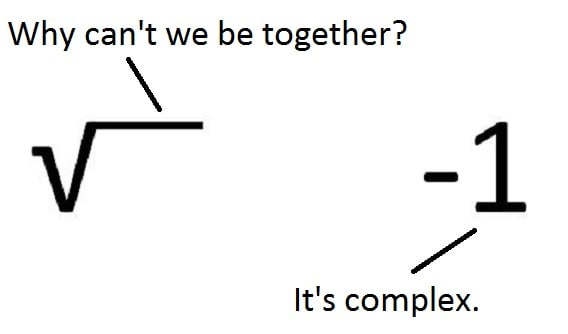# Operations with Complex NumbersIn the previous section we’ve discussed complex numbers, considered how the concept arose and have given some basic ideas about how to deal with this stuff. In this section we’re going to consider how to perform basic operations with complex numbers. This time we’ll go through every detail and show several examples. We’ll start with addition.

## Addition of complex numbers

Suppose we have two complex numbers $z_1$ and $z_2$ and we want to add them: $$z_1=5+3i$$ $$z_2=2-4i$$ We treat these numbers as algebraic expressions when adding. Grouping real and imaginary numbers we obtain: $$z_1+z_2=5+3i+2-4i=(5+2)+(3i-4i)=7-i$$ Subtraction is performed in similar way: $$z_1-z_2=5+3i-(2-4i)=5+3i-2+4i=3+7i$$ As you could notice, numbers of different nature – real and imaginary – are added and subtracted separately: real numbers go with real numbers, and imaginary numbers go with imaginary numbers. We cannot dazzle real and imaginary numbers to obtain a single value of either kind. That’s just like we cannot dazzle money of two currencies without conversion.

Find more math and physics tutorials on our youtube channel.

## Multiplication of complex numbers

Let’s multiply $z_1$ and $z_2$. Again we’re treating them as algebraic expressions. Also we need to recall that $i^2=-1$ and here’s what we obtain: $$z_1\cdot z_2=(5+3i)(2-4i)=10-20i+6i-12i^2=10-14i+12=22-14i$$ As you may already notice, when we multiply a real number by an imaginary number, the result is imaginary, like in our example $5\cdot (-4i)=-20i$. If we multiply an imaginary number by a real number, the result is real, as for $3i\cdot (-4i)=-12i^2=12$.

## Division of complex numbers

Complex conjugates

Unlike operations we’ve already discussed – addition, subtraction and multiplication – division is not performed that obviously. First we need to discuss complex conjugates a little bit. Suppose we have a complex number given in general form: $$z=x+iy$$ Its complex conjugate is denoted as $\bar{z}$ and equals: $$\bar{z}=x-iy$$ The sign in front of imaginary part is changed, as you can see from the formula for complex conjugate. Important property of complex conjugates is that their product is a real number. Let’s check it by multiplying $z$ and $\bar{z}$: $$z\cdot \bar{z}=(x+iy)(x-iy)=x^2-x\cdot iy+iy\cdot x-iy\cdot iy=x^2-ixy+ixy-y^2i^2=x^2+y^2$$ Thus, product of complex conjugates is indeed a real number. That’s a good thing to keep in mind. Now we’re ready to discuss division. Return to our initial pair of complex numbers $z_1$ and $z_2$. Let’s divide $z_1$ by $z_2$. First we write it as a fraction: $$z_1\div z_2=\frac{z_1}{z_2}= \frac{5+3i}{2-4i}$$ This is not yet the answer since we cannot tell what’s the real and imaginary part of such number. In order to be able to distinguish them we need to somehow get rid of imaginary numbers in the denominator. And that’s where complex conjugates come in handy. The trick here is that we multiply the fraction’s numerator and denominator by complex conjugate of denominator, thus obtaining real number at the bottom. Complex conjugate of $z_2$ is $\bar{z_2}=2+4i$. Now we multiply numerator and denominator of our fraction by $2+4i$:

\begin{aligned}\frac{5+3i}{2-4i}=\frac{(5+3i)(2+4i)}{(2-4i)(2+4i)}=\frac{10+20i+6i+12i^2}{4+6i-6i-16i^2}=\frac{10+26i-12}{4+16}=\frac{-2+26i}{20}&\\=\frac{-2}{20}+\frac{26i}{20}=-\frac{1}{10}+\frac{13}{10}i\end{aligned}

And this is our result with now obvious real and imaginary part. Let’s consider another example. This time we’ll divide a complex number by its complex conjugate to check if there’s anything special about this case: \begin{aligned}z_1\div \bar{z_1}=\frac{z_1}{\bar{z_1}}=\frac{5+3i}{5-3i}=\frac{(5+3i)(5+3i)}{(5-3i)(5+3i)}=\frac{25+15i+15i+9i^2}{25+9}=\frac{25+30i-9}{34}=&\\\frac{16+30i}{34}=\frac{16}{34}+\frac{30}{34}i=\frac{8}{17}+\frac{15}{17}i\end{aligned} As you can see, there’s nothing special about dividing a complex number by its complex conjugate. Thus, we’ve considered how to add, subtract, multiply and divide complex numbers, what’s complex conjugate and how it comes in handy. At the beginning we considered a complex number in general form $z=x+iy$. This is called algebraic form of complex number and is not the only way to represent it. In the next section we’ll discuss other approaches (trigonometric, exponential) and also consider geometric representation of complex numbers.2 Shares
Filed under Math.